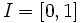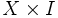# Paracompact Hausdorff implies binormal

Jump to: navigation, search
This article gives the statement and possibly, proof, of an implication relation between two topological space properties. That is, it states that every topological space satisfying the first topological space property (i.e., paracompact Hausdorff space) must also satisfy the second topological space property (i.e., binormal space)
View all topological space property implications | View all topological space property non-implications
Get more facts about paracompact Hausdorff space|Get more facts about binormal space

## Statement

Any paracompact Hausdorff space (i.e., a space that is both paracompact and Hausdorff) is a binormal space.

## Definitions used

### Paracompact Hausdorff space

Further information: paracompact Hausdorff space

A topological space$X$ is termed a paracompact Hausdorff space if it satisfies the following two conditions:

1. It is a paracompact space: every open cover has a locally finite open refinement.
2. It is a Hausdorff space: any two points can be separated by disjoint open subsets.

### Binormal space

Further information: binormal space

A topological space$X$ is termed a binormal space if the product of$X$ and the unit interval$[0,1]$ (equipped with the Euclidean topology) is a normal space.

## Proof

Given: A paracompact Hausdorff space$X$.$I = [0,1]$ is the unit interval.

To prove: The space$X \times I$ is a normal space.

Proof:

1.$X \times I$ is paracompact: This follows from$X$ being paracompact,$I$ being compact, and fact (1).
2.$X \times I$ is Hausdorff: This follows from fact (2).
3.$X \times I$ is normal: The previous two steps yield that$X \times I$ is paracompact Hausdorff. Fact (3) now yields that$X \times I$ is normal.